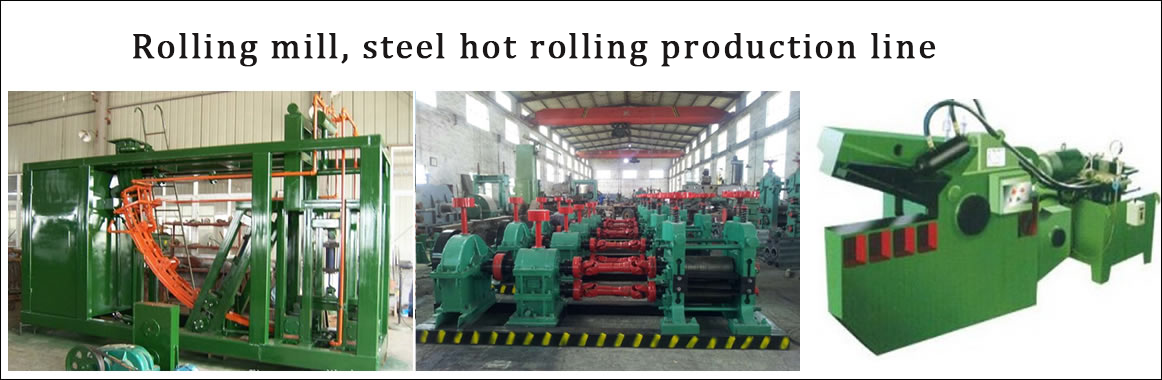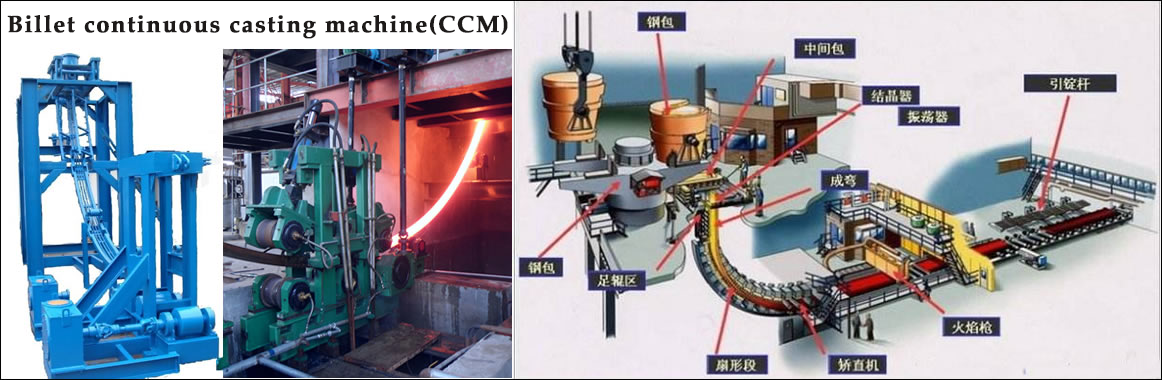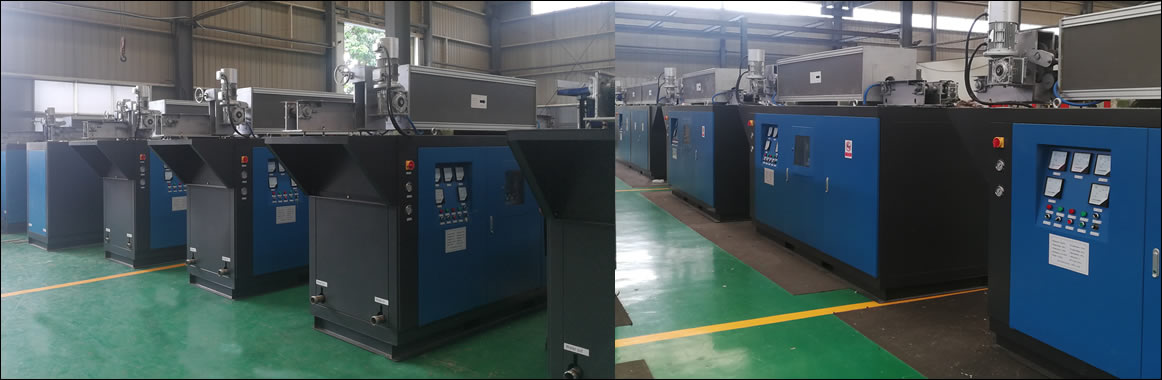••••••Contact
Tel: 18037961302
Sales hot line ( 24 hours  service): 18037961302

E-Mail: firstfurnace@gmail.com

whatsapp:+8618037961302

Adress: Luoxin Industrial Park, Luoyang, Henan
Electric furnace technical information
Selection of main components of medium frequency power supply for induction heating furnace
1, the calculation of the parameters of the thyristor
The power of the steel tube heating furnace is 1500 KW, and the design incoming line voltage is 500V. The following data can be obtained by calculation.
DC voltage Ud=1.35×500=675V
DC current Id=1500000÷675=2200A
IF voltage US=1.5×Ud=1000V
Rectifier silicon rated current IF = 0.38 × Id ÷ 2 ÷ 0.85 = 491A
(The division by 2 is because there are two sets of identical rectification parts)
Rectified silicon rated voltage UV=1.414×UL=1.414×500=707V
Inverter silicon rated current IF=Id/2=1100A
Inverter silicon rated voltage UV = 1.414 × US = 1414V
2, SCR model selection plan
The rectifier thyristor is KP1500A/2000V, that is, the rated current is 1500A, and the rated voltage is 2000V. Compared with the theoretical value, the voltage margin is 2.26 times and the current margin is 2.43 times.
The inverter thyristor KK2500A/2000V of the intermediate frequency power supply, that is, the rated current is 2500A, and the rated voltage is 2000V. Moreover, the inverter silicon is connected to the inverter bridge by means of a double silicon series connection, so that the actual rated voltage of the thyristor on each inverter bridge arm is 5000V. Compared with the theoretical value, the voltage margin is 2.26 times and the current margin is 2.15 times.
3, intermediate frequency resonant capacitor cabinet
The intermediate frequency resonant capacitors of this capacitor cabinet are all selected from the electric capacitors produced by Xinanjiang Power Capacitor Factory, and the model is RFM2 1.0 -2000-1.0S. Its capacity is 2000KVar and its frequency of use is 1000Hz.